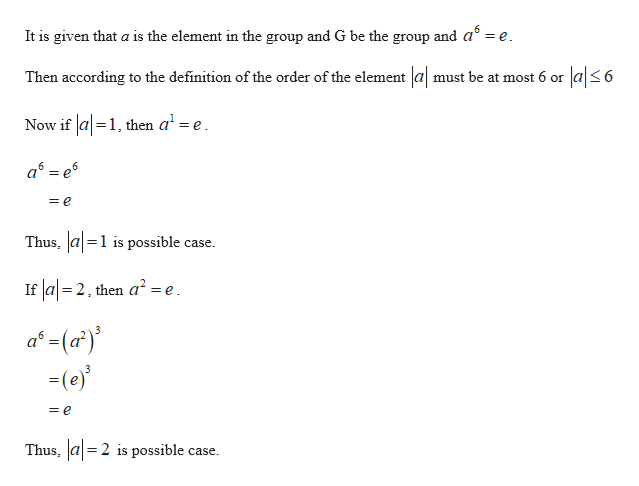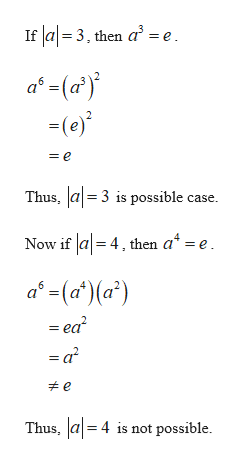Suppose that a is a group element and a^6 = e. What are the possibilities for |a|? Provide reasons for your answer.

Question

Suppose that is a group element and a^6 = e. What are the possibilities for |a|? Provide reasons for your answer.

Step 1

It is known that if G be a group and a be any element of group G, then n  is said to be order of a if n is the least positive integer such that an = e, the identity element of G.

From the definition it can be concluded that if m is any positive integer such that am = e, then |a| ≤ m.help_outlineImage TranscriptioncloseIt is given that a is the element in the group and G be the group and a =e Then according to the definition of the order of the element |a| must be at most 6 or a|6 Now if a1, then a' =e a e = e Thus, a1 is possible case If a 2, then a2 =e a-(a) (e) 3 = e Thus, a2 is possible case = fullscreen
Step 2help_outlineImage TranscriptioncloseIf a3, then a = e -(e) = e Thus, a3 is possible case. Now if la 4, then a* = e a(a')(a) - ea? e Thus, a4 is not possible. fullscreen

Want to see the full answer?

See Solution

Want to see this answer and more?

Our solutions are written by experts, many with advanced degrees, and available 24/7

See Solution
Tagged in

Math